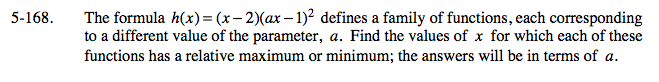### Home > CALC > Chapter 5 > Lesson 5.5.2 > Problem5-168

5-168.Notice that h(x) is a cubic function. Visualize its graph.

consequently, it is the location of a local max or min of h(x).

Since h(x) is a cubic function, there should be another local max or min. Find its location by setting h '(x) = 0 and solving for x.

Recall that maxima and minima are y-values. So evaluate h(x) to find the corresponding y-values.